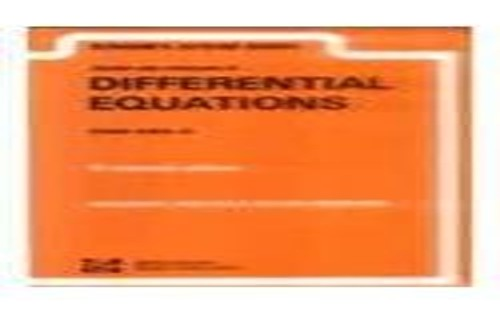# Schaum's Outline of Differential Equations by Frank Ayres

Out of Stock
\$25.99
inc. GST
N/A
Only 0 left

## Schaum's Outline of Differential Equations Summary

### Schaum's Outline of Differential Equations by Frank Ayres

Unfortunately we do not have a summary for this item at the moment

### Why buy from World of BooksOur excellent value books literally don't cost the earthFree delivery in AustraliaEvery used book bought is one saved from landfill

McGraw-Hill authors represent the leading experts in their fields and are dedicated to improving the lives, careers, and interests of readers worldwide

Origin of Differential Equations.Solutions of Differential Equations.Equations of First Order and First Degree.Variables Separable; Exact Equations.Linear Equations.Geometric Applications.Physical Applications.Equations of First Order and Higher Degree.Singular Solutions.Extraneous Loci.Applications of First Order and Higher Degree Equations.Linear Equations of Order n.Homogeneous Linear Equations with Constant Coefficients.Linear Equations with Constant Coefficients.Short Methods.Linear Equations with Variable Coeffiecients--The Cauchy and Legendre Linear Equations.Linear Equations with Variable Coefficient--Equations of the Second Order.Linear Equations with Variable Coefficients--Miscellaneous Types.Applications of Linear Equations.Simultaneous Linear Equations.Total Differential Equations.Applications.Numerical Approximations.Integration in Series.The Legendre, Bessel, and Gauss Equations.Partial Differential Equations.Linear Partial Differential Equations of Order One.Non-Linear Partial of Order One.Homogeneous Partial of Higher Order with Constant Coefficients.Non-Homogeneous Linear Equations with Constant Coefficients.Partial Differential Equations of Order Two with Variable Coefficients.